# Bearing fault feature extraction method based on complete ensemble empirical mode decomposition with adaptive noise

## Maohua Xiao1, Cunyi Zhang2, Kai Wen3, Longfei Xiong4, Guosheng Geng5, Dan Wu6

1, 2, 3, 4, 5, 6College of Engineering, Nanjing Agricultural University, Nanjing, 210031, China

1Corresponding author

Journal of Vibroengineering, Vol. 20, Issue 7, 2018, p. 2622-2631. https://doi.org/10.21595/jve.2018.19562
Received 22 December 2017; received in revised form 26 April 2018; accepted 15 June 2018; published 15 November 2018

Copyright © 2018 Maohua Xiao, et al. This is an open access article distributed under the Creative Commons Attribution License, which permits unrestricted use, distribution, and reproduction in any medium, provided the original work is properly cited.
Views 179
Abstract.

As an important part of rotating machinery, bearings play an important role in large-scale mechanical equipment. Abnormal bearing conditions may cause the machine to malfunction, or even evolve into a serious accident. Therefore, the accurate and timely fault diagnosis of the bearing is of great significance. Based on EMD, this paper introduces the working principles and characteristics of EEMD and CEEMDAN, respectively. Then the signal was decomposed by EEMD and CEEMDAN respectively. The simulation results show that CEEMDAN has better effect on signal decomposition. Then, comparing the effect of CEEMDAN and EEMD on bearing fault feature frequency extraction, the experiment proves that CEEMDAN has a better ability to preserve original signal and eliminate noise than EEMD method, and can extract bearing fault feature more accurately and timely.

Keywords: CEEMDAN, EEMD, denoising, fault diagnosis, feature extraction, bearings.

#### 1. Introduction

As an important part of rotating machinery, bearings play an important role in large-scale mechanical equipment. However, due to the harsh working environment of the bearing, including corrosion, poor lubrication and plastic deformation, the bearing becomes one of the most easily broken parts of the machine. In order to diagnose bearing failures in a timely manner and prevent unpredictable accidents, it is particularly important to study bearing fault feature extraction techniques. In engineering applications, the fault characteristic frequency of the bearing is often extracted from the collected vibration signal. Therefore, vibration signal processing technology is widely used in the industrial field as the main analysis technology for bearing fault diagnosis.

EMD is a new time-frequency analysis method proposed by Huang, which can decompose the signal into several intrinsic modal functions (IMFs) [1, 2]. The method can perform adaptive selection of base at the same time when multi-resolution decomposition is completed. This method solves the problem of base selection and is suitable for handling nonlinear and non-stationary signals. However, mode mixing may occur when decomposing the intrinsic mode component (IMC) [3, 4]. In order to reduce the mode mixing, Wu Z. et al. proposed the Ensemble Empirical Mode Decomposition (EEMD), which reduces the effect of mode mixing by adding auxiliary white noise and achieves good results to a certain extent [5, 6]. Zheng J. et al. proposed a partly ensemble EMD (PEEMD) method to solve the problem of mode mixing, which is an improvement on EEMD . However, EEDM also has the problems of low decomposition efficiency, too many pseudo-components, and ineffective extraction of fault features.

In order to solve these problems of the EEMD, Torres M. E. et al. proposed the complete ensemble empirical mode decomposition with adaptive noise (CEEMDAN) method with adaptive noise . This method adds adaptive white noise in each decomposition stage to reduce the mode mixing. Colominas M. A. et al. used the IMF of Gaussian white noise decomposed by EMD as the unique noise to be added at each decomposition stage to solve the “false” IMFs that may be generated after CEEMDAN decomposes the signal . Lei Y. et al. also applied this method to the fault diagnosis of locomotive rolling bearings . But the diagnostic efficiency of this method was greatly reduced. This paper describes the use of CEEMDAN in bearing feature extraction and uses experiments to compare the accuracy of the proposed method with EEMD, and verifies the feasibility of the proposed method [11, 12].

The rest of this study is organized as follows. Section 2 presents the basic principles of EEMD and CEEMDAN. Section 3 describes and compares the simulation of EEMD and CEEMDAN. Section 4 provides an empirical comparison of the feature extraction between EEMD and CEEMDAN. Section 5 presents a concluding summary.

#### 2.1. EEMD’s principles

EMD is based on the time scale characteristics of complex signal itself without any requirement to setup any basis functions . This method decomposes noise signals into a series of data sequence retaining local feature information, which is called Intrinsic Mode Function (IMF). Based on this characteristic, EMD has a high signal-to-noise ratio while dealing with non-stationary and nonlinear data. EMD method needs to meet the following 3 conditions:

(1) At least one maxima and one minimum must be contained in the signal.

(2) Time characteristic scale is defined by the time interval between adjacent extreme points.

(3) If there is no extreme point in the whole signal, but a turning point is available. Then differential can be done to the signal to obtain the extreme points. Finally, obtain the final decomposition results through the integration of IMFs.

The EEMD method is improved on the basis of the EMD method. The mode mixing phenomenon will frequently appear while using EMD method. The reasons are as the following due to two kinds of circumstances:

(1) A separate IMF signal contains completely different scale.

(2) The same scale appears in different IMFs. The EEMD method used the statistic characteristic that white Gaussian noise has a uniform frequency distribution. After additional Gauss white noise background is added in the signal, signal region at different scales will be automatically mapped to the appropriate scale of background white noise. This makes it possible to have a unified framework for the analysis of time scales which can separate various time scales clearly, enhance the anti-mixing effect and form an effective, adaptive, and dynamic two filter group, solving the mode mixing problem of EMD method.

The specific process of EEMD method:

(1) Add Gauss white noise with zero mean and variance as constant $\omega \left(t\right)$ on the original signal $\gamma \left(t\right)$ and get a new target signal $Y\left(t\right)$:

(1)
$Υ\left(t\right)=\gamma \left(t\right)+\omega \left(t\right).$

(2) Imply EMD decomposition on the signal $Y\left(t\right)$. Obtained A series of IMF components and residual components ${r}_{Ν}\left(t\right)$ of the target signal:

(2)
$Υ\left(t\right)={\sum }_{j=1}^{N}im{f}_{j}\left(t\right)+{r}_{N}\left(t\right).$

(3) Add $N$ sub different white noise to the target signal, and repeat the steps above:

(3)
${Υ}_{i}\left(t\right)={\sum }_{j=1}^{N}im{f}_{ij}\left(t\right)+{r}_{iN}\left(t\right).$

(4) Do overall average operation of the above results to eliminate the influence of the many times adding of Gauss white noise on real IMF. Get the IMF component:

(4)
$im{f}_{j}\left(t\right)=\frac{1}{n}{\sum }_{i=1}^{n}im{f}_{ij}\left(t\right).$

#### 2.2. CEEMDAN’s principle

EEMD has great significance in solving the problem of mode mixing in EMD. But it has several drawbacks. First, a large amount of computation is needed. Second, many false components will be decomposed and achieved if the parameter chosen is not reasonable. Torres M E and others put forward CEEMDAN method to solve this problem. This method adds adaptive white noise in every decomposing stage and calculates the only left residual signal to achieve every model component . In this way, the problem of EEMD was solved.

The specific process of CEEMDAN method:

(1) Defined operator ${Ε}_{k}\left(\bullet \right)$ to represents the first $k$ modal component generated by the EMD calculation.$\omega \left(t\right)$ meet the Gauss white noise of $N$ (0, 1). $\stackrel{-}{{IMF}_{k}}$ is denoted as the first $k$ modal component of the CEEMDAN algorithm. Let $Y\left[t\right]$ be the signal to be processed. CEEMDAN decomposed the signals $Y\left[t\right]+{\mathcal{E}}_{0}{\omega }_{i}\left(t\right)$, using EMD, for $I$ times, and obtained:

(5)
${\stackrel{-}{IMF}}_{1}=\frac{1}{I}{\sum }_{i=1}^{I}{\stackrel{-}{IMF}}_{i1}.$

(2) Calculate the first margin of the first phase of the ($k=$ 1):

(6)
${Υ}_{1}\left[t\right]=Y\left[t\right]-{\stackrel{-}{IMF}}_{1}.$

(3) Discompose these signals ${Υ}_{1}\left[t\right]+{\epsilon }_{1}{Ε}_{1}\left({\omega }_{i}\left[t\right]\right)$ ($i=$ 1, 2, …, $I\right)$, till the first EMD mode component is decomposed. Then defined the second modal component as:

(7)
${\stackrel{-}{IMF}}_{2}=\frac{1}{I}{\sum }_{i=1}^{I}{E}_{1}\left({Υ}_{1}\left[t\right]+{\epsilon }_{1}{E}_{1}\left({\omega }_{i}\left[t\right]\right)\right).$

(4) While $k=$ 2, …, $K$, calculate the first $K$ margin:

(8)
${Υ}_{k}\left[t\right]={Υ}_{k-1}\left[t\right]-{\stackrel{-}{IMF}}_{k}.$

(5) Discompose these signals ${Υ}_{k}\left[t\right]+{\epsilon }_{k}{Ε}_{k}\left({\omega }_{i}\left[t\right]\right)$ ($i=$ 1, 2, …, $I$), till the first EMD mode component is decomposed. Then defined the $K+$ 1 modal components as:

(9)
${\stackrel{-}{IMF}}_{k+1}=\frac{1}{I}{\sum }_{i=1}^{I}{E}_{1}\left({Υ}_{k}\left[t\right]+{\epsilon }_{k}{E}_{k}\left({\omega }_{i}\left[t\right]\right)\right).$

(6) Add 1 to $K$, continue to carry out step 4 till the residual signal achieved can’t be decomposed any more (every residual signal contains on more than one extreme point). Finally, $K$ intrinsic mode functions are obtained. Residual signals meet:

(10)

#### 3. Simulation

Harmonics are resonant waves with the same frequency or multiple frequencies as the main wave! They are parasitized with the main wave! It is commonly known as harmonics and higher harmonics! In order to verify CEEMDAN’s better denoising effect, this paper strictly set the same harmonic signals: 50 Hz, 150 Hz, and 300 Hz for simulation experiments. To better compare the decomposition performance of CEEMDAN and EEMD on the signal, set the same input parameters for the two methods, that is, the white noise amplitude is 0.1, the ensemble size is 100, and the sampling frequency is 2000 Hz, to ensure that the simulation verification is more accurate. The sampling time is 2 s and $i\left(t\right)$ is the gause white noise signal, as shown in Eq. (11):

(11)
$x\left(t\right)=2\mathrm{sin}\left(100\pi t\right)+3\mathrm{cos}\left(300\pi t\right)+\mathrm{sin}\left(600\pi t\right)+i\left(t\right).$

The modal components are obtained by the decomposition of signals. However, some pseudo modal components are often obtained and not associated with original signals. So, these pseudo modal components in modal components need to be identified and eliminated. Calculate the correlation coefficient between each modal component and the original signal and remove the pseudo modal component. The formula of the correlation coefficient is following:

(12)
${r}_{XY}=\frac{{\sum }_{i=1}^{N}\left({X}_{i}-\stackrel{-}{X}\right)\left({Y}_{i}-\stackrel{-}{Y}\right)}{\sqrt{{\sum }_{i=1}^{N}{\left({X}_{i}-\stackrel{-}{X}\right)}^{2}}\sqrt{{\sum }_{i=1}^{N}{\left({Y}_{i}-\stackrel{-}{Y}\right)}^{2}}}.$

Fig. 1 shows the correlation coefficients between the first 8 modes of EEMD decomposition and the original signal. Obviously, the correlation coefficient of the first four modes is much larger than that of other modes. So, extract the first four modal components and reconstruct them. This eliminates residual modal components in the signal.

Fig. 1. The correlation coefficient between the first 8 modes decomposed by EEMD and original signal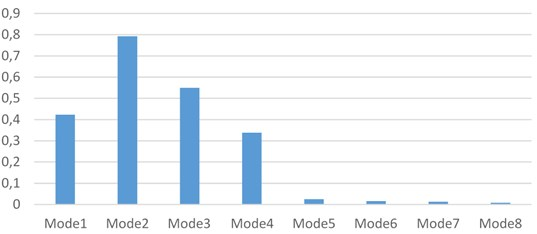Fig. 2. The correlation coefficient between the first 8 modes decomposed by CEEMDAN and original signal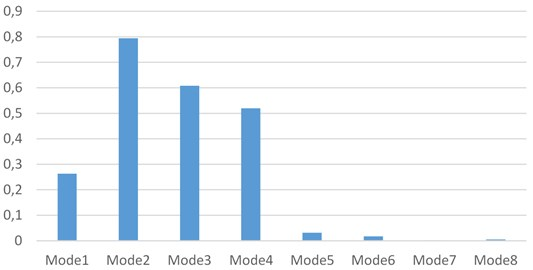Fig. 2 shows the correlation coefficients between the first 8 modes of the CEEMDAN decomposition and the original signal. In the same way, the first four modal components are extracted and reconstructed to eliminate residual modal components in the signal.

The signals were decomposed using EEMD and CEEMDAN, respectively. Then calculate the correlation coefficient between the reconstructed signal and the original signal. The signal-noise ratio reflects the purity of the signal, the larger the SNR, the stronger the signal purity. The signal-to-noise ratio of the reconstructed signal is calculated by the following equation:

(13)
${S}_{NR}=10×{\mathrm{log}}_{10}\left\{\sum _{i=1}^{N}\frac{{{S}_{i}}^{2}}{\sum _{i=1}^{N}{\left({S}_{i}-{{S}_{i}}^{\text{'}}\right)}^{2}}\right\}.$

Table 1 shows the correlation coefficient between the original signal and the reconstructed signal processed by the two methods, and also shows the signal-to-noise ratio of the reconstructed signal processed by the two methods.

Table 1. The correlation coefficient and signal-to-noise ratio

 Processed by EEMD Processed by CEEMDAN Correlation coefficient 0.9769 0.9774 Signal to noise ratio 13.1768 13.2108

Table 1 shows the correlation coefficient between the original signal and the reconstructed signal processed by the two methods.

From Table 1, it can be concluded that the proposed method can effectively remove the interference factors in the signal and the resulting reconstructed signal can better reflect the original signal.

#### 4. Feature extraction

To verify the effectiveness of this method, this paper chooses the rolling bearing as the research object then process the vibration signal of the bearing outer ring and the inner ring fault respectively.

Bearing outer ring fault characteristic frequency expression is:

(14)
${f}_{o}=\frac{1}{2}\left(1-\frac{d}{{D}_{m}}\mathrm{c}\mathrm{o}\mathrm{s}\alpha \right){f}_{n}Z.$

Bearing inner ring fault characteristic frequency expression is:

(15)
${f}_{i}=\frac{1}{2}\left(1+\frac{d}{{D}_{m}}\mathrm{c}\mathrm{o}\mathrm{s}\alpha \right){f}_{n}Z.$

In the equation, $Ζ$ represents the number of rolling elements, d presents the diameter of rolling body, a represents the bearing contact angle, ${D}_{m}$ represents the pitch circle diameter of bearing and ${f}_{n}$ presents rotating frequency.

This paper uses bearing data measured by fault simulation test platform of rolling bearing in Electrical Engineering Laboratory of Case Western Reserve University.

The platform’s parameters are set as follows:

Bearing type is 6205-2RS JEM SKF, bearing speed is 1797 r/min, the sampling frequency is 12 kHz, the number of data points is 4096, and fault diameter set is 0.021 inches. Measuring points are arranged in the drive motor output of the platform.

The following Table 2 shows the fault frequency of the bearing outer ring and the inner ring calculated by the Eq. (14) and the Eq. (15).

In Fig. 3, the time domain waveform and spectrum of the fault signal of the inner ring are presented, when the bearing’s rotational speed is at 1797 rpm. And from Fig. 3, it is appreciated that the spectrum cannot identify directly the fault frequency mixed with a variety of noise signals.

Table 2. Bearing fault characteristic frequency

 Rotational frequency ${f}_{n}$ Outer ring fault frequency ${f}_{0}$ Inner fault frequency ${f}_{i}$ 29.95 Hz 107.36 Hz 162.18 Hz

Fig. 3. The time domain waveform and spectrum of the fault signal of the inner ring at 1797 rpm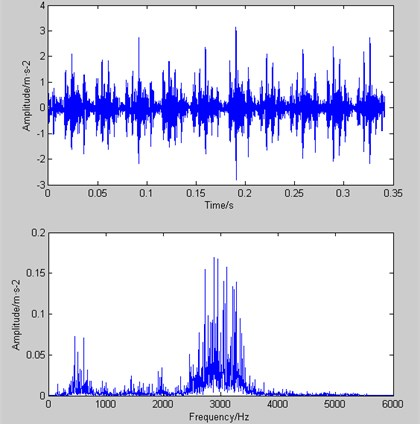In the case of decomposing the inner ring’s fault signal through EEMD and CEEMDAN separately, the total modal number of CEEMDAN, both are 12, doesn’t have any increase compared with EEMD. Usually, the several modes in front, produced by extraction, are chosen as research objects because their correlation coefficients with original signals are high. Therefore, the paper takes the first 4 modes of EEMD and CEEMDAN respectively as the research objects. Which are shown in Fig. 4 and Fig. 5.

Fig. 4. The first 4 modes of EEMD (the inner ring)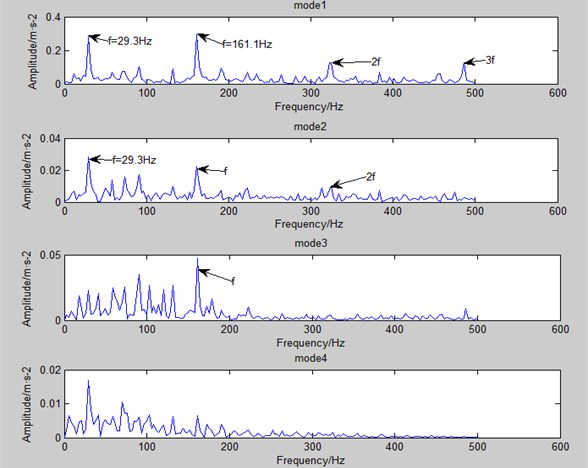Fig. 5. The first 4 modes of CEEMDAN (the inner ring)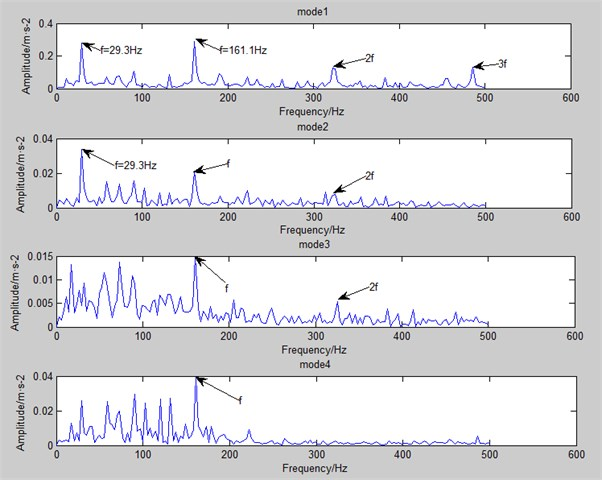In Fig. 4 and Fig. 5, the frequency around 29.5 Hz is bearing rotational frequency, and that around 162 Hz is of inner ring fault. What’s more, 2$f$ frequency can also be seen in the figure, which is equal to 2 times the fault frequency, and 3$f$ etc. it can be found obviously that CEEMDAN can find out more fault frequency and multiple frequency (especially in mode 3 and mode 4).

In addition, the paper uses the decomposed spectrum to analyse the case of outer ring. In Fig. 6, the time domain waveform and spectrum of the fault signal of the outer ring are presented, when the bearing's rotational speed is at 1797 rpm. As it can be seen, the fault frequency mixed with a variety of noise signals cannot be identified directly.

Fig. 6. The time domain waveform and spectrum of the fault signal of the outer ring at 1797 rpm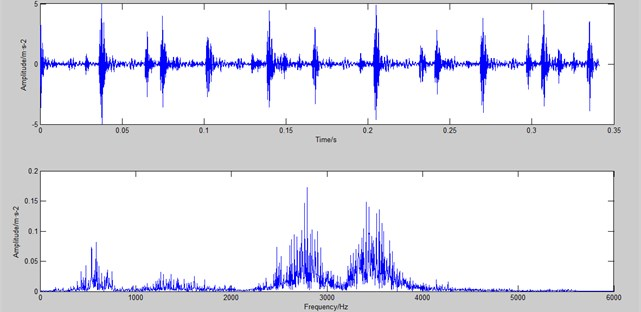As is shown in Fig. 7 and Fig. 8, EEMD and CEEMDAN is applied to decompose the outer ring fault signal separately. EEMD produces 12 modes, and CEEMDAN produces 13 modes. The total modal number of CEEMDAN is only 8 % more than EEMD and there isn’t any obvious increase. Just like the case in inner ring’s spectrum. The several modes in front have higher correlation coefficient with original signals. Therefore, the paper takes the first 3 modes of CEEMDAN and EEMD respectively as the research objects.

In spectrum above, the frequency around 29.5 Hz is bearing rotational frequency, and that around 108 Hz is of outer ring fault. What’s more, it can be seen 2$f$ frequency, 3$f$ frequency etc. It is easy to find that CEEMDAN can find out more fault frequency and multiple frequency (especially in mode 3).

In conclusion, CEEMDAN is more effective than EEMD in extracting the characteristic frequencies of the fault signals inside and outside the bearing. Multiple modal components processed by CEEMDAN can identify the failure frequency of the signal. However, signals processed by EEMD are prone to mode mixing.

Fig. 7. The first 3 modes of EEMD (the outer ring)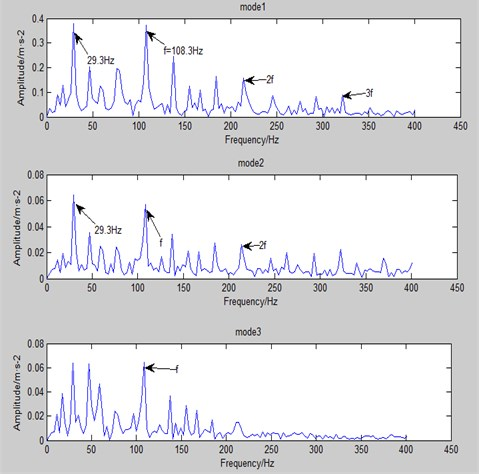Fig. 8. The first 3 modes of CEEMDAN (the outer ring)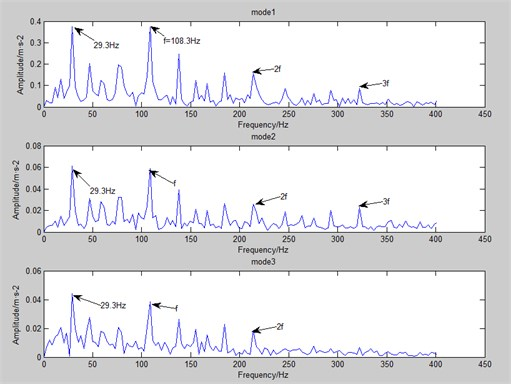#### 5. Conclusions

Based on the EMD, this paper describes in detail the working principles and features of EEMD and CEEMDAN. The feature extraction method in bearing fault diagnosis was analyzed. Through simulation experiments and bearing fault feature extraction experiments, this paper verifies that CEEMDAN has great advantages in retaining original signal information and eliminating signal noise. As a new technology to study bearing fault feature extraction, CEEMDAN has important research value and application prospects.

#### Acknowledgements

The research is funded partially by the National Key Research and Development Program of China (2016YFD0701103) and the China Postdoctoral Science Foundation (2016M601800).

1. Huang N., Shen Z., Long S., Wu M., Shih H., Zheng Q., Yen N., Tung C., Liu H. The empirical mode decomposition and the Hilbert spectrum for nonlinear and non-stationary time series analysis. Proceedings of The Royal Society A, Vol. 454, 1998, p. 903-995. [Publisher]
2. Hao F., Xu G., Huang X. Explosive NQR signal processing based on empirical mode decomposition and wavelet transform. Vibration and Shock, Vol. 16, 2014, p. 183-187. [Search CrossRef]
3. Liu Z., Sun W., Zeng J. A new short-term load forecasting method of power system based on EEMD and SS-PSO. Neural Computing and Applications, Vol. 24, Issues 3-4, 2014, p. 973-983. [Publisher]
4. Singh D. S., Zhao Q. Pseudo-fault signal assisted EMD for fault detection and isolation in rotating machines. Mechanical Systems and Signal Processing, Vol. 81, 2016, p. 202-218. [Publisher]
5. Wu Z., Huang N. E. Ensemble empirical mode decomposition: a noise-assisted data analysis method. Advances in Adaptive Data Analysis, 2009, https://doi.org/10.1142/S1793536909000047. [Publisher]
6. Wu Z., Huang N. E., Chen X. The multi-dimensional ensemble empirical mode decomposition method. Advances in Adaptive Data Analysis, Vol. 1, Issue 3, 2009, p. 339-372. [Publisher]
7. Zheng J., Cheng J., Yang Y. Partly ensemble empirical mode decomposition: an improved noise-assisted method for eliminating mode mixing. Signal Processing, Vol. 96, 2014, p. 362-374. [Publisher]
8. Torres M. E., Colominas M. A., Schlotthauer G., et al. A complete ensemble empirical mode decomposition with adaptive noise. IEEE International Conference on Acoustics, Speech and Signal Processing, 2011, p. 4144-4147. [Search CrossRef]
9. Colominas M. A., Schlotthauer G., Torres M. E. Improved complete ensemble EMD: A suitable tool for biomedical signal processing. Biomedical Signal Processing and Control, Vol. 14, 2014, p. 19-29. [Publisher]
10. Lei Y., Liu Z., Ouazri J., et al. A fault diagnosis method of rolling element bearings based on CEEMDAN. Proceedings of the Institution of Mechanical Engineers, Part C: Journal of Mechanical Engineering Science, Vol. 231, Issue 10, 2017, p. 1804-1815. [Search CrossRef]
11. Xu X., Jiang Y., Pueh L. Multi-objective optimal design of sandwich panels using a genetic algorithm. Engineering Optimization, Vol. 49, Issue 10, 2017, p. 1665-1684. [Publisher]
12. Xu X., Jiang Y., Pueh L., Chen N. Sound insulation performance optimization of lightweight sandwich panels. Journal of Vibroengineering, Vol. 18, Issue 4, 2016, p. 2574-2586. [Publisher]
13. Satish M., Karunesh K., Kota S. Vibro-acoustic fault analysis of bearing using FFT, EMD, EEMD and CEEMDAN and their implications. Advances in Machine Learning and Signal Processing, Vol. 387, 2016, p. 281-292. [Publisher]
14. Bouhalais M., Djebala A., Ouelaa N., et al. CEEMDAN and OWMRA as a hybrid method for rolling bearing fault diagnosis under variable speed. International Journal of Advanced Manufacturing Technology, Vol. 94, Issues 5-8, 2018, p. 2475-2489. [Publisher]

#### Cited By

 World Electric Vehicle Journal Guangxin Li, Yong Chen, Wenqing Wang, Yimin Wu, Rui Liu 2022 International Journal of Mechanical Sciences Suchao Xie, Yaxin Li, Hongchuang Tan, Runda Liu, Fengyi Zhang 2022 Complexity Helong Yu, Kang Yuan, Wenshu Li, Nannan Zhao, Weibin Chen, Changcheng Huang, Huiling Chen, Mingjing Wang, Narayanan Kumarappan 2021 Energies Saidatul Habsah Asman, Nur Fadilah Ab Aziz, Ungku Anisa Ungku Amirulddin, Mohd Zainal Abidin Ab Kadir 2021 Zhenjin Shi, Yueyang Li, Shuai Liu 2020